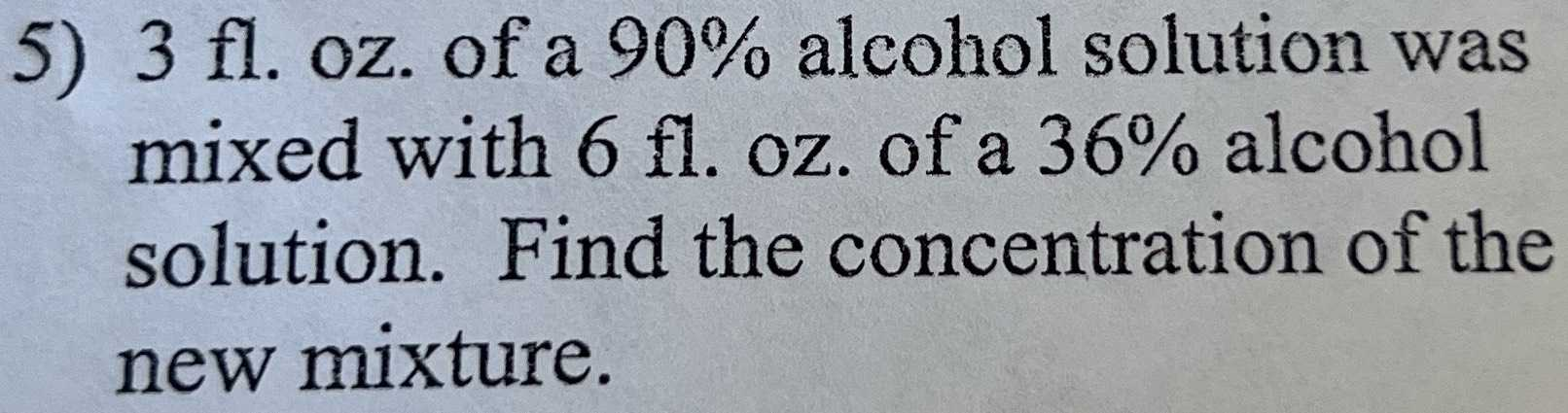### Still have math questions?$$3$$ fl. oz. of a $$90 \%$$ alcohol solution was mixed with $$6 fl$$ . oz. of a $$36 \%$$ alcohol solution. Find the concentration of the new mixture.
$$\frac { 3\times 90\% + 6\times 36\% } { 3+ 6} = 65.1\%$$